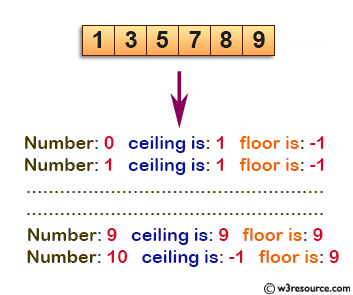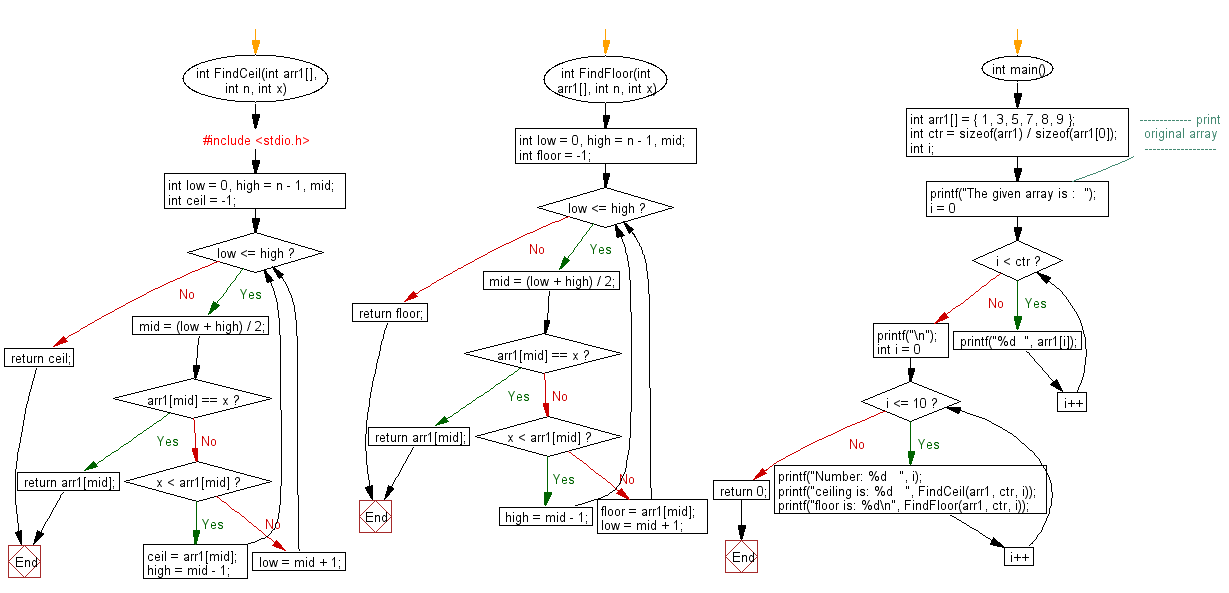﻿ C exercises: Find the Floor and Ceil of the number 0 to 10 from a sorted array - w3resource# C Exercises: Find the Floor and Ceil of the number 0 to 10 from a sorted array

## C Array: Exercise-41 with Solution

Write a program in C to find the Floor and Ceil of the number 0 to 10 from a sorted array.

Pictorial Presentation:Sample Solution:

C Code:

``````#include <stdio.h>
int FindCeil(int arr1[], int n, int x)
{
int low = 0, high = n - 1, mid;
int ceil = -1;
while (low <= high)
{
mid = (low + high) / 2;
if (arr1[mid] == x)
return arr1[mid];
else if (x < arr1[mid])
{
ceil = arr1[mid];
high = mid - 1;
}
else
low = mid + 1;
}
return ceil;
}

int FindFloor(int arr1[], int n, int x)
{
int low = 0, high = n - 1, mid;
int floor = -1;
while (low <= high)
{
mid = (low + high) / 2;
if (arr1[mid] == x)
return arr1[mid];
else if (x < arr1[mid])
high = mid - 1;
else
{
floor = arr1[mid];
low = mid + 1;
}
}
return floor;
}
int main()
{
int arr1[] = { 1, 3, 5, 7, 8, 9 };
int ctr = sizeof(arr1) / sizeof(arr1);
int i;
//------------- print original array ------------------
printf("The given array is :  ");
for(i = 0; i < ctr; i++)
{
printf("%d  ", arr1[i]);
}
printf("\n");
//--------------------------------------------

for (int i = 0; i <= 10; i++)
{
printf("Number: %d   ", i);
printf("ceiling is: %d   ", FindCeil(arr1, ctr, i));
printf("floor is: %d\n", FindFloor(arr1, ctr, i));
}
return 0;
}
```
```

Sample Output:

```The given array is :  1  3  5  7  8  9
Number: 0   ceiling is: 1   floor is: -1
Number: 1   ceiling is: 1   floor is: 1
Number: 2   ceiling is: 3   floor is: 1
Number: 3   ceiling is: 3   floor is: 3
Number: 4   ceiling is: 5   floor is: 3
Number: 5   ceiling is: 5   floor is: 5
Number: 6   ceiling is: 7   floor is: 5
Number: 7   ceiling is: 7   floor is: 7
Number: 8   ceiling is: 8   floor is: 8
Number: 9   ceiling is: 9   floor is: 9
Number: 10   ceiling is: -1   floor is: 9
```

Flowchart:C Programming Code Editor:

Improve this sample solution and post your code through Disqus.

What is the difficulty level of this exercise?

﻿